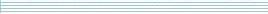### Counting & Sampling Contingency Tables

Speaker
Dr. M. Cryan
Duration
1:01:27
Date
22 April 2009
Abstract
Suppose we are given two lists r and c of positive integers, where r=(r,...., r[m]) represents a list of prescribed row sums and c=(c, ..., c[n]) is a list of prescribed column sums. We require that (r + ... + r[m]) =(c + ... + c[n]). In this setting, we say that a m-by-n matrix X of non-negative integers is a Contingency Table (for these given row/column values) if X simultaneously satisfies all of the given row and column sums. The problem of determining whether at least one contingency table exists can be solved in polynomial-time (in fact, this question is fairly trivial).

In my talk, we are interested in the more-difficult problem of randomly sampling a table uniformly at random, from the entire set of contingency tables. This problem has some applications in practical statistics which I will mention. We study a very natural Markov chain on the set of contingency tables called the 2-by-2 heat bath: one step of this chain operates by selecting 2 rows and 2 columns uniformly at random, computing the induced row sums and column sums on this 2-by-2 window, then replacing the window with a table chosen randomly from all 2-by-2 tables with the induced row and column sums. This Markov chain converges to the uniform distribution on contingency tables - our goal is to show that it approaches uniformity within polynomial-time. We are able to achieve this result for the case when the number of rows m is some fixed constant. Our proof is by application of the canonical paths method of Jerrum and Sinclair.

(Joint work with Martin Dyer, Leslie Goldberg, Mark Jerrum and Russell Martin)
You should see the video here…
High quality
Fullscreen
Volume